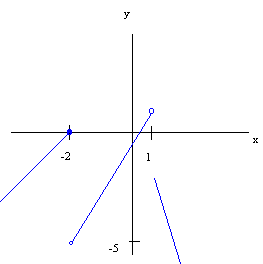$$\newcommand{\id}{\mathrm{id}}$$ $$\newcommand{\Span}{\mathrm{span}}$$ $$\newcommand{\kernel}{\mathrm{null}\,}$$ $$\newcommand{\range}{\mathrm{range}\,}$$ $$\newcommand{\RealPart}{\mathrm{Re}}$$ $$\newcommand{\ImaginaryPart}{\mathrm{Im}}$$ $$\newcommand{\Argument}{\mathrm{Arg}}$$ $$\newcommand{\norm}{\| #1 \|}$$ $$\newcommand{\inner}{\langle #1, #2 \rangle}$$ $$\newcommand{\Span}{\mathrm{span}}$$

# 2.1: Lines

$$\newcommand{\vecs}{\overset { \rightharpoonup} {\mathbf{#1}} }$$ $$\newcommand{\vecd}{\overset{-\!-\!\rightharpoonup}{\vphantom{a}\smash {#1}}}$$$$\newcommand{\id}{\mathrm{id}}$$ $$\newcommand{\Span}{\mathrm{span}}$$ $$\newcommand{\kernel}{\mathrm{null}\,}$$ $$\newcommand{\range}{\mathrm{range}\,}$$ $$\newcommand{\RealPart}{\mathrm{Re}}$$ $$\newcommand{\ImaginaryPart}{\mathrm{Im}}$$ $$\newcommand{\Argument}{\mathrm{Arg}}$$ $$\newcommand{\norm}{\| #1 \|}$$ $$\newcommand{\inner}{\langle #1, #2 \rangle}$$ $$\newcommand{\Span}{\mathrm{span}}$$ $$\newcommand{\id}{\mathrm{id}}$$ $$\newcommand{\Span}{\mathrm{span}}$$ $$\newcommand{\kernel}{\mathrm{null}\,}$$ $$\newcommand{\range}{\mathrm{range}\,}$$ $$\newcommand{\RealPart}{\mathrm{Re}}$$ $$\newcommand{\ImaginaryPart}{\mathrm{Im}}$$ $$\newcommand{\Argument}{\mathrm{Arg}}$$ $$\newcommand{\norm}{\| #1 \|}$$ $$\newcommand{\inner}{\langle #1, #2 \rangle}$$ $$\newcommand{\Span}{\mathrm{span}}$$

## 1. Lines (definitions)

Everyone knows what a line is, but providing a rigorous definition proves to be a challenge.

Definition: Line

A line with slope $$m$$ through a point $$P = (a,b)$$ is the set of all points $$(x,y)$$ such that

$\dfrac{y-b}{x-a}= m.$

## 2. The Slope Intercept Form of the equation of a Line

Given a point $$(x_1,y_1)$$ and a slope $$m$$, the equation of the line is

Definition: Slope Intercept Equation of a Line

$y-y_1=m(x-x_1)$

## 3. Piecewise Linear Functions

A function is piecewise linear if it is made up of parts of lines

Example 1

$f(x)=\begin{cases} x+4 & \text{if }x\leq-2 \\ 2x-1 & \text{if } -2<x<1 \\ -2x & \text{if } x\geq1\end{cases}$We graph this line by sketching the appropriate parts of each line on the same graph.

## 4. Applications

Example 2

Suppose you own a hotel that has 150 rooms. At $80 per room, you have 140 rooms occupied and for every$5 increase in price you expect to have two additional vacancies. Come up with an equation that gives rooms occupied as a function of price.

Solution

Let $$x$$ be the price of a room and $$y$$ be the number of rooms occupied. Then we have an equation of a line that passes through the point $$(80,140)$$ and has slope $$-\frac{1}{5}$$. Hence the equation is:

$y - 140 = -\dfrac{1}{5}(x - 80)$

or

$y = -\dfrac{1}{5} x + 16 + 140$

or

$y = -\dfrac{1}{5} x + 156.$

Exercise 1

What should you do if your two year old daughter has a 40 degree C temperature?

Hint: We have the two points: $$(0,32)$$ and $$(100,212)$$.

Exercise 2

Suppose that your company earned $30,000 five years ago and$35,000 three years ago. Assuming a linear growth model, how much will it earn this year?

Exercise 3

My rental was bought for $204,000 three years ago. Depreciation is set so that the house depreciates linearly to zero in twenty years from the purchase of the house. If I plan to sell the house in twelve years for$250,000 and capital gains taxes are 28% of the difference between the purchase price and the depreciated value, what will my taxes be?

Exercise 4

Wasabi restaurant must pay either a flat rate of \$400 for rent or 5% of the revenue, whichever is larger. Come up with the equation of the function that relates rent as a function of revenue

Larry Green (Lake Tahoe Community College)